Integral of sec²(x)|Two ways

Hi everyone! I know it’s been a really long time since my last post, but lessons during COVID-19 really had me busy and stressed out… but here I am again! Hope you are all doing great and ready for more integrals!Graph of the function f(x)=sec²(x) in red and the F(x) in blue.

In this post I am going to show you two methods I found to integrate the function y=sec²x. The first one requires a bit of knowledge about trigonometry and trigonometric identities and might not be very intuitive, whereas the second involves a substitution right at the beginning and then some algebra and trigonometric identities. Feel free to share more methods if you know them!

First method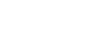First of all, what is sec(x) equal to? It’s simply the reciprocal of the function cos(x), i.e. 1/cos(x). Therefore, secant squared is equal to one over cosine squared. That simple!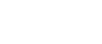Now comes the not very intuitive part. In trigonometry, 1 is given by the sum of the squares of the two fundamental functions sine and cosine. This is the most important trigonometric identity and the one we are going to use: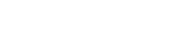At this point we basically have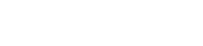It’s like (a+b)*(1/c)=a/c+b/c: we are simply distributing the 1/c.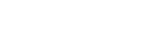Let’s apply linearity:Remember the property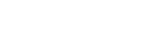where m is a constant, in our case 1; therefore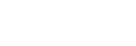The problem here is mostly the fact that we have sine squared at the numerator. If it was simply sin(x), we would have performed a u-substitution by letting u=cos(x) and its derivative, -sin(x), would have gone to the denominator and so it would have cancelled out with the sin(x) on top.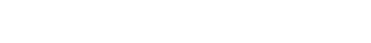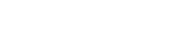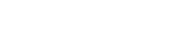Notice that cos²(x) becomes u² because we let u=cos(x).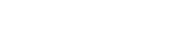Let’s apply the power rule for integrals. Just in case you don’t remember,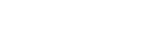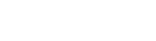Let’s undo the substitution (remember: u=cos(x))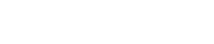BUT we don’t have this integral; instead we haveBut after all, sin²(x) is sin(x)*sin(x), so: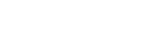We have a product, which means we can perform integration by parts, and naturally we are going to differentiate sin(x) and integrate the other term, since we have already found the antiderivative (1/cos(x));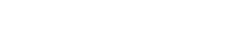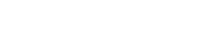Remember that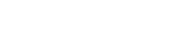Therefore,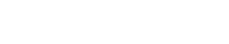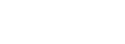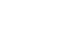This is the result of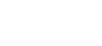but we also have that x from the first part of the integration: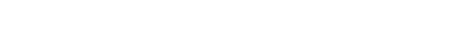sin(x)/cos(x) is tan(x); finally,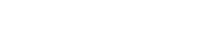Second methodThis time we are going to let u be sec(x), so that sec²(x) becomes u²: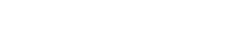sec(x) is 1/cos(x), so in order to differentiate it we apply the reciprocal rule: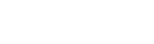Therefore,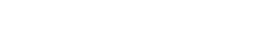Now, there is a problem: if we substitute we must integrate with respect to the new variable, u in our case, which means every function of the “old” variable x must be written in terms of u. We have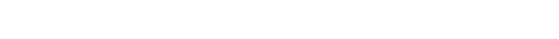The terms in red are simply u²: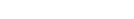We’re almost there. How can we write sin(x) using cos²(x)? Remember the identity sin²(x)+cos²(x)=1: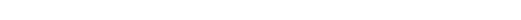We know that u²=1/cos²(x), therefore cos²(x)=1/u²: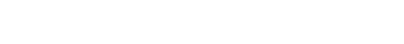This means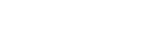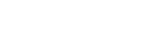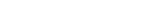Let’s give a look at the integral: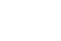We want dx, therefore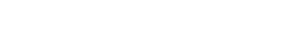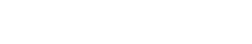This is exactly what we wanted, no more x‘s. At this point what do you think we can do? Give it a try and then keep reading to see if you got it right.

This is what we are going to do: if you differentiate u²-1, you’ll get 2u, which will be the du but, since we want dx, it will “become” 1/2u and so it will cancel out with the u at the numerator. Since we used u before, we’ll change variable.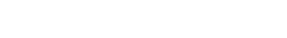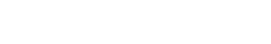1/2 is a constant and is moved in front of the integral; at this point we can apply the power rule for integrals: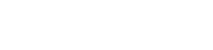We are almost done; let’s undo all substitutions. Remember: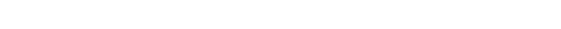That’s the “final” result; “final” because we can actually do more: sec²(x)-1 comes from the trigonometric identity sec²(x)-tan²(x)=1 and, as you can see, it is equal to tan²(x); this means that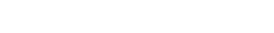Finally,That’it! We’ve integrated the function sec²(x) with two methods. If you weren’t able to do it by yourself, keep in mind that looking at the procedures is actually a great way to learn the mechanism and all of the techniques; this is how I started, so stay positive!

I really hope you found this post helpful and if you enjoyed it leave a like and subscribe for more! In the next post we’ll find the integral of csc²(x), so in the mean time, give it a try!

Rating: 5 out of 5.

Join 31 other subscribers.

{{#message}}{{{message}}}{{/message}}{{^message}}It appears your submission was successful. Even though the server responded OK, it is possible the submission was not processed. Please contact the developer of this form processor to improve this message. Learn more{{/message}}

Submitting…

Posts you may find useful

Integration techniques | U substitution

Ok, we now have the basis of integration! As I said in the previous post, I am now going to cover the techniques of integration. I will make a post for each method, because it’s important that you understand each of them before you can move on. In this lesson I teach you how to… Read more Integration techniques | U substitutionIntegration techniques | U substitution – part 2

U-substitution is the most common technique used in integration. It can happen, however, that it doesn’t work out, no matter what you try to substitute. You may think of using another technique, like integration by parts, but it’s not always necessary. You realise u-sub is not working when you still see one or more x’s.… Read more Integration techniques | U substitution – part 2Integration techniques | Integration by parts

U-substitution is a very useful technique for integration. You can apply it in every case, but it doesn’t always make things easier. But here comes this new technique in help! Integration by parts is used to calculate the integral of products. Well, everything is actually a product, (x² for example is x*x but also x²*1)… Read more Integration techniques | Integration by partsRules and properties of integrals

Ok, this is the second step! I will cover the rules you need to apply to calculate an integral. With the next lesson, you will hopefully be able to start integrating! Let’s start! But first, I recommend you check out my post “What’s integration?” https://addjustabitofpi.com/2019/09/28/whats-integration/ Linearity The sum or difference of two (or more) functions… Read more Rules and properties of integralsThe rules of differentiation

In the previous lesson we talked about differentiation in general; just as a reminder, it is a word that means “computation of a derivative”, the same way multiplication means “computation of a product” . Calculating the derivative of a function is quite easy if you know the rules of differentiation, which I will explain in… Read more The rules of differentiation